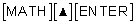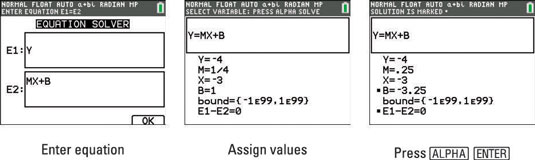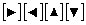##### TI-84 Plus CE Graphing Calculator For Dummies, 3rd EditionDid you know that your TI-84 Plus calculator can solve equations that have more than one variable? The trick is that you must assign values to all the variables except the one that you’re solving for. For example, here is a classic math question:

Find the equation of the line (in slope-intercept form) that goes through the point (3, 4) and has a slope of 1/4.

To solve this problem, enter the equation Y = M*X + B into the Solver. To access the Solver, pressEnter the equation into E1 and E2. See the first screen.After you have entered an equation in the Solver, the values assigned to the variables in your equation are the values that are currently stored in those variables in your calculator. You must assign an accurate value to all variables except the variable you’re solving for. These values must be real numbers or arithmetic expressions that simplify to real numbers.

To assign a value to a variable, use thekeys to place the cursor on the number currently assigned to that variable and then key in the new value. As you start to key in the new value, the old value is erased. Press [ENTER] when you’re finished entering the new value (as illustrated in the second screen, where values are assigned to variables Y, M, and X).

Enter a guess for B and press [ALPHA][ENTER] to solve the equation. Since you have found the value of B, you can answer the question and write the equation of the line, Y = 1/4X 13/4.